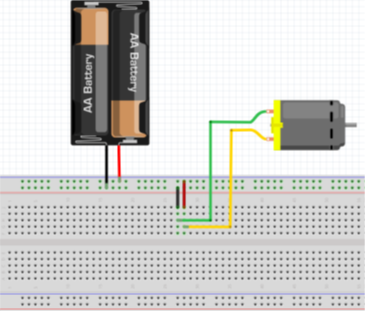## Electric Vehicle Basics

Assume in the following circuit we have a 12V battery and the resistance across the motor when running without a load is 40 ohms.
1. How much current, in mA, will flow through the motor?
2. What is the power, in watts, dissipated by the motor?
3. If the motor ran for 10 minutes under the above conditions, what is the minimum battery capacity in mAh?Hint
$$Power=voltage \times current$$$Hint 2 Ohm's Law $$V=iR$$$
where $$V$$ is the voltage, $$i$$ is the current, and $$R$$ is the resistance.
To solve for the current, use Ohm's law:
$$V=IR$$$where $$V$$ is the voltage, $$I$$ is the current, and $$R$$ is the resistance. $$I=\frac{V}{R}=\frac{12V}{40\Omega }=0.3A=300\:mA$$$
Power absorbed by a resistive element:
$$P=VI$$$where $$P$$ is power, $$V$$ is the voltage, and $$I$$ is the current. Solving for power dissipated by the motor: $$P=12V\times 0.3A=3.6\:W$$$
To solve for capacity:
$$300mA \times 10min \times\frac{1h}{60min}=50\:mAh$$\$
Current = 300 mA
Power = 3.6 W
Capacity = 50 mAh